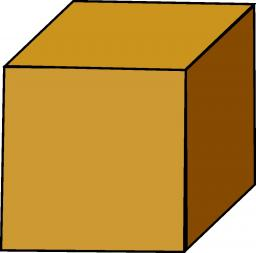# Heavier 4553

What is the mass of a cube of cast iron with an edge length of 2.3 dm if we know that the mass of 1 dm³ of cast iron is 7.3 kg? Will the same size cube of cork be heavier if we know that the weight of 1 m³ of cork is 250 kg?

m1 =  88.8191 kg
m2 =  3.0418 kg

### Step-by-step explanation:Did you find an error or inaccuracy? Feel free to write us. Thank you!

Tips for related online calculators
Do you know the volume and unit volume, and want to convert volume units?